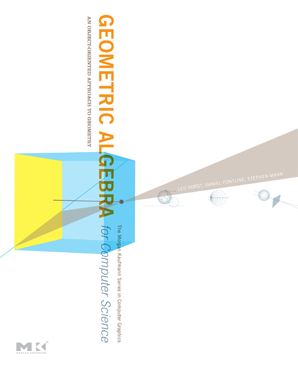This is the companion site to the book Geometric Algebra For Computer Science, An Object Oriented Approach to Geometry, published by Morgan Kaufmann Publishers.
Here you can find a preview and tour of the book, and download extra content. We provide interactive versions of almost all figures in the book, source code for the programming exercises, some of the answers to the structural exercises, and we correct errors in the book.Geometric algebra is a consistent computational framework for geometric programming. It has new, geometrically meaningful products to calculate directly with the subspaces of a vector space. This capability considerably reinforces and extends the linear algebra techniques traditionally used in computer graphics and robotics. It naturally integrates other useful frameworks (such as complex numbers, quaternions and Plücker coordinates.) into real geometry.

The result is a high-level specification language for geometrical operations that can be executed directly. Constructions that used to require matrices or coordinate manipulations can now be expressed immediately in terms of the geometric quantities that define them. Moreover, since all this exists within one unified framework, no processing time is wasted on data conversions from one mathematical system to another.

A powerful new feature of geometric algebra is a 5D operational model of 3D Euclidean geometry. It makes lines, planes, circles, spheres and tangents basic elements of computation, and represents general rigid body motions by `rotors'. These real operators extend the two-sided quaternion computations to deal with all motions (rather than just rotations through the origin). They can be universally applied to any of the elements, and are easily interpolated.

This book consists of three parts. Part I extends linear algebra to geometric algebra, focusing on the new products and constructions involved in making an algebra of subspaces and their operators. These chapters are self-contained (they only require elementary knowledge of linear algebra) and fairly mathematical, but richly illustrated to develop the intuition in tandem with the representational skills. Part II uses geometric algebra to model geometries, culminating in the powerful conformal model with its universally applicable Euclidean operators. Part III consists of implementational techniques useful in applications, with a ray tracer fully worked out.

Features

• Explains GA both as a natural extension of linear algebra and in terms of its significance for 3D programming in graphics, vision and robotics.
• Systematically explores the concepts and techniques that are key to representing basic objects and geometric operators using GA.
• Covers in detail the conformal model, a convenient way to implement 3D geometry using a 5D representation space.
• Presents effective approaches to making GA an integral part of your programming.
• Includes numerous drills and programming exercises helpful for both students and practitioners.
• This companion web site includes interactive figures, answers to exercises, and downloadable sofware for the instructive programming exercises that conclude each chapter.

This book can be used for a graduate course (or advanced undergraduate course - basic linear algebra and a reasonable level of mathematical sophistication is sufficient background for most of the text) in computer science, combining useful mathematics with applications in robotics and computer graphics. It is also suitable for self-study in the continued education of professionals in the fields requiring geometric modeling, such as robotics, computer vision, graphics and computer games.